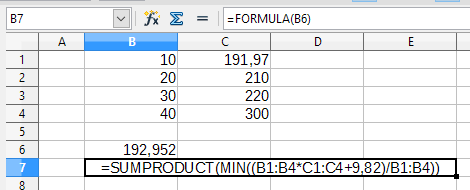# calculate minimum sale price of stocks

I have the following table (see image).
Here I want to calculate (simplified) the minimum sale price.
Currently I just devide the balance through the amount of stocks.
The balance itself is calculated by adding the sum of all efforts and proceeds.
If I’m not wrong this results in a min selling price that garantuees no overall loss (ignoring selling fees).

Problem is of course you want to take away your profit and calculate the selling price on the resulting rest.
So in this easy example I would want to get:

`(10 * \$191.97 + 9.82)/10 = \$192.952`

How do I calculate this (in LibreOffice Calc)?
(of course without calculating it just manually on that row)Perhaps something like:I fear it is not that easy. What if you wanted to calculate the min selling price after the sale of the 45 units on 01/09/15. After that 7 units were left. How would you calculate the min selling price there. You need to take fees into account then too but figuring them out is not that easy at that point.

That is different of your question. If you want someone helping, share a clear example file with what you want achieve.

That’s why I said it is an easy example. But just an example. Of course you would want to calculate the min selling price at any point no matter how many sales of buys you did.